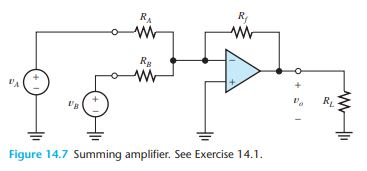# Question Solved1 AnswerExercise 14.1 A circuit known as a summer is shown in Figure 14.7. a. Use the ideal- op-amp assumption to solve for the output voltage in terms of the input voltages and resistor values. b. What is the input resistance seen by VA? c. By va? d. What is the output resistance seen by RL?YJZLWJ The Asker · Electrical EngineeringTranscribed Image Text: Exercise 14.1 A circuit known as a summer is shown in Figure 14.7. a. Use the ideal- op-amp assumption to solve for the output voltage in terms of the input voltages and resistor values. b. What is the input resistance seen by VA? c. By va? d. What is the output resistance seen by RL?
More
Transcribed Image Text: Exercise 14.1 A circuit known as a summer is shown in Figure 14.7. a. Use the ideal- op-amp assumption to solve for the output voltage in terms of the input voltages and resistor values. b. What is the input resistance seen by VA? c. By va? d. What is the output resistance seen by RL?